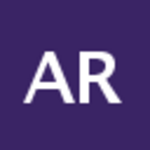Community Profile# Abinaya

Active since 2014

#### Statistics

••#### Content Feed

View by

Question

connecting points in Binary image
In a binary image i have points and i need to connect the points in that image. How it cn be done?

meer dan 8 jaar ago | 2 answers | 0

### 2

Question

How to draw polygon having set of unordered points?
How to draw polygon having set of unordered points in binary image.I want approximately all the points to be connected in the po...

meer dan 8 jaar ago | 1 answer | 0

### 1

Question

how to make qhull file operable
I am trying to apply skeletonisation to voronoi diagram. http://www.mathworks.in/matlabcentral/fileexchange/27543-skeletonizatio...

meer dan 8 jaar ago | 2 answers | 0

### 2

Question

Skeletonisation of an image
in my project i have applied voronoi diagram . next sterp is applying skeletonisation. I came to know that skeletonisation is ap...

meer dan 8 jaar ago | 1 answer | 0

### 1

Question

extracting the road pattern from satellite images
My project work is extracting the road pattern from images. I applied houghlines to detect the lines and got the start and end p...

meer dan 8 jaar ago | 0 answers | 0

### 0

Question

skeletonisation algorithm using voronoi diagram
have some roads and others binary images and I have its voronoi diagram of the image. I'm trying to calculate the skeleton.. not...

meer dan 8 jaar ago | 2 answers | 0

### 2

Question

Voronoi diagram to skeletonisation
How to get the skeleton of an image having its delaunay triangulation and voronoi diagram.Please help me out with matlab code. A...

meer dan 8 jaar ago | 1 answer | 0

### 1

Question

Is it possible to apply skeletonisation to voronoi
Can anyone please tell me what is the concept of skeletonisation.. Is it possible to apply skeletonisation to voronoi diagram? P...

meer dan 8 jaar ago | 1 answer | 0

### 1

Question

how to find the area of the triangles formed as a result of delaunay triangulation
I have applied delaunay triangulation to my image. i have got 160 triangles. i need to find area of each triangle. Kindly help m...

meer dan 8 jaar ago | 2 answers | 0

### 2

Question

Delaunay triangulation output . How to find vertices after applying triangulation
After applying triangulation, how to get the 1) vertices of triangles 2) area of triangles 3) length of the edges.. Pleas...

meer dan 8 jaar ago | 0 answers | 0

### 0

Question

After applying delaunay triangulation how to find area of those triangles??
I have applied delaunay triangulation after detecting lines.. I have the vertices of the triangles. Help me with matlab code to...

meer dan 8 jaar ago | 1 answer | 0

### 1

Question

beginner's question regarding plotting the start and end points of the lines detected
% Plot beginnings and ends of lines plot(xy(1,1),xy(1,2),'.','LineWidth',2,'Color','yellow'); start(k,1) = xy(1,1); ...

meer dan 8 jaar ago | 2 answers | 0

### 2

Question

Beginner's question- correction in matlab code for road detection from satellite images project
for k = 1:length(lines) len = norm(lines(k).point1 - lines(k).point2); if (len > minlinelen) xy = [lines(k).point1...

meer dan 8 jaar ago | 0 answers | 0

### 0

Question

How to detect curved lines
how will you find peaks for curve line and also give coding for line coding for connecting that curve peaks. Please help me

meer dan 8 jaar ago | 2 answers | 0

### 2

Question

Plotting & Clustering points in Matlab needed clarification
<</matlabcentral/answers/uploaded_files/7851/output.jpg>> In this figure the lines are detected.We are asked to plot the red ...

meer dan 8 jaar ago | 1 answer | 0

### 1

Question

Matlab program lines need explanation
Peaks = houghpeaks(H,40,'threshold',ceil(0.3*max(H(:)))); x = theta(Peaks(:,2)); y = rho(Peaks(:,1)); plot(x,y,'...

meer dan 8 jaar ago | 0 answers | 0

### 0

Question

Line detection //contour tracing/plot...pls help needed in matlab
For the given image the lines are detected using hough transform.What should be done to connect these lines?? Pls help me with...

meer dan 8 jaar ago | 1 answer | 0

### 1

Question

Mask an image to avoid line detection in that regions.
how to mask certain portions in an image.We have to detect roads from satellite images. We also get regions covered with trees a...

meer dan 8 jaar ago | 1 answer | 0

### 1

Question

Curve detection in roads help needed in the matlab code
I am doing a project of roadline extraction. I have detected the lines using hough transform. But the curves and turns in road g...

meer dan 8 jaar ago | 0 answers | 0